﻿ Python: Find the second smallest number in a list - w3resource# Python: Find the second smallest number in a list

## Python List: Exercise - 27 with Solution

Write a Python program to find the second smallest number in a list.

Example - 1 :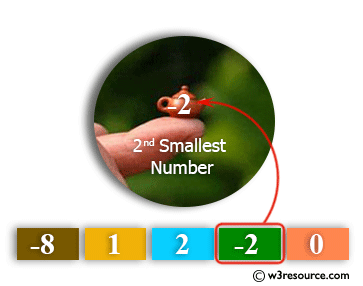Example - 2 :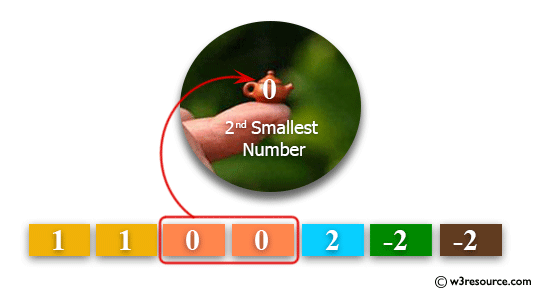Example - 3 :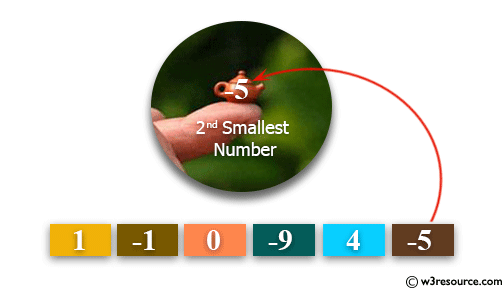Example - 4 :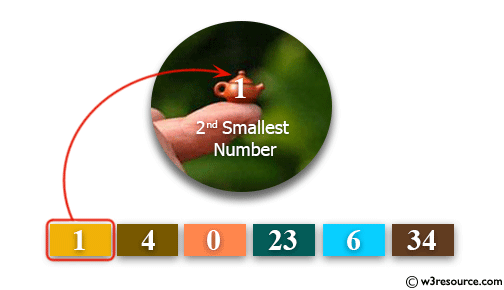Sample Solution:-

Python Code:

``````def second_smallest(numbers):
if (len(numbers)<2):
return
if ((len(numbers)==2)  and (numbers == numbers) ):
return
dup_items = set()
uniq_items = []
for x in numbers:
if x not in dup_items:
uniq_items.append(x)
uniq_items.sort()
return  uniq_items

print(second_smallest([1, 2, -8, -2, 0, -2]))
print(second_smallest([1, 1, 0, 0, 2, -2, -2]))
print(second_smallest([1, 1, 1, 0, 0, 0, 2, -2, -2]))
print(second_smallest([2,2]))
print(second_smallest())
```
```

Sample Output:

```-2
0
0
None
None
```

Flowchart: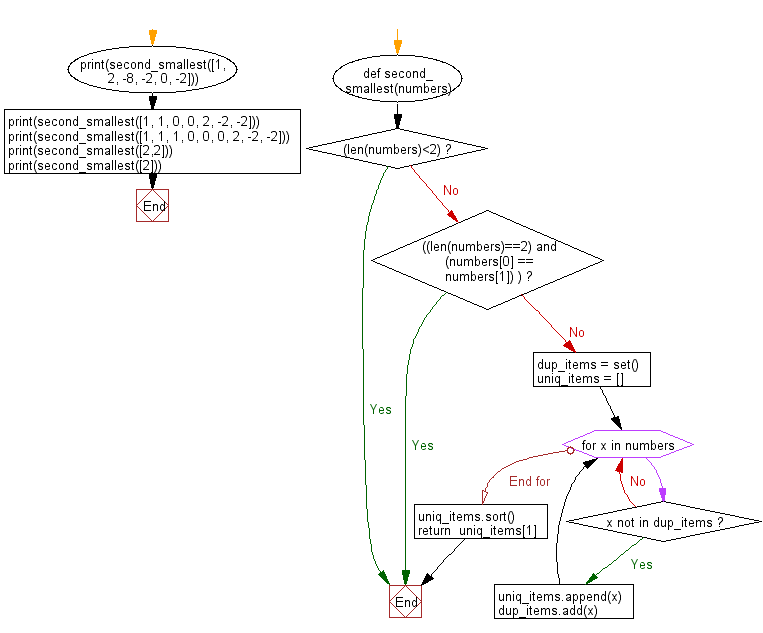## Visualize Python code execution:

The following tool visualize what the computer is doing step-by-step as it executes the said program:

Python Code Editor:

Have another way to solve this solution? Contribute your code (and comments) through Disqus.

What is the difficulty level of this exercise?

Test your Python skills with w3resource's quiz

﻿

## Python: Tips of the Day

```print(2_000_000)
```2000000